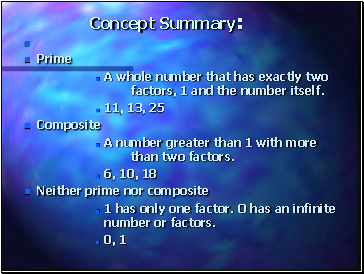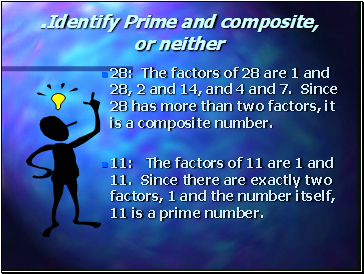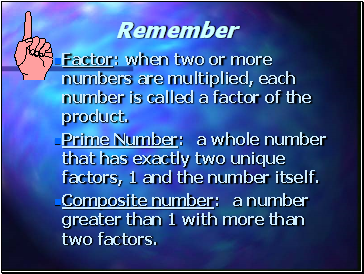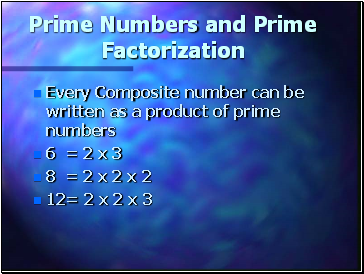# Prime FactorizationPage 1

#### WATCH ALL SLIDES

Slide 1Prime Factorization by Dr. Emerlina C. Binuya

every composite number expressed as a product of a prime number.

Slide 2## Concept Summary

Prime

A whole number that has exactly two factors, 1 and the number itself.

11, 13, 25

Composite

A number greater than 1 with more than two factors.

6, 10, 18

Neither prime nor composite

1 has only one factor. O has an infinite number or factors.

0, 1

Slide 3## Identify Prime and composite, or neither

28: The factors of 28 are 1 and 28, 2 and 14, and 4 and 7. Since 28 has more than two factors, it is a composite number.

11: The factors of 11 are 1 and 11. Since there are exactly two factors, 1 and the number itself, 11 is a prime number.

Slide 4## Remember

Factor: when two or more numbers are multiplied, each number is called a factor of the product.

Prime Number: a whole number that has exactly two unique factors, 1 and the number itself.

Composite number: a number greater than 1 with more than two factors.

Slide 5## Prime Numbers and Prime Factorization

Every Composite number can be written as a product of prime numbers

6 = 2 x 3

8 = 2 x 2 x 2

12= 2 x 2 x 3

Slide 6## Prime Factors

Factors that are prime numbers are called prime factors. You can use Factor Tree to find prime factors. This one shows the prime factors of 50

50

2

25

5

5

50=2 x 5 x 5 is the prime factorization of 50.

Slide 7Hope you enjoy the Lesson!

Have a nice day to all of you!!!!!!!